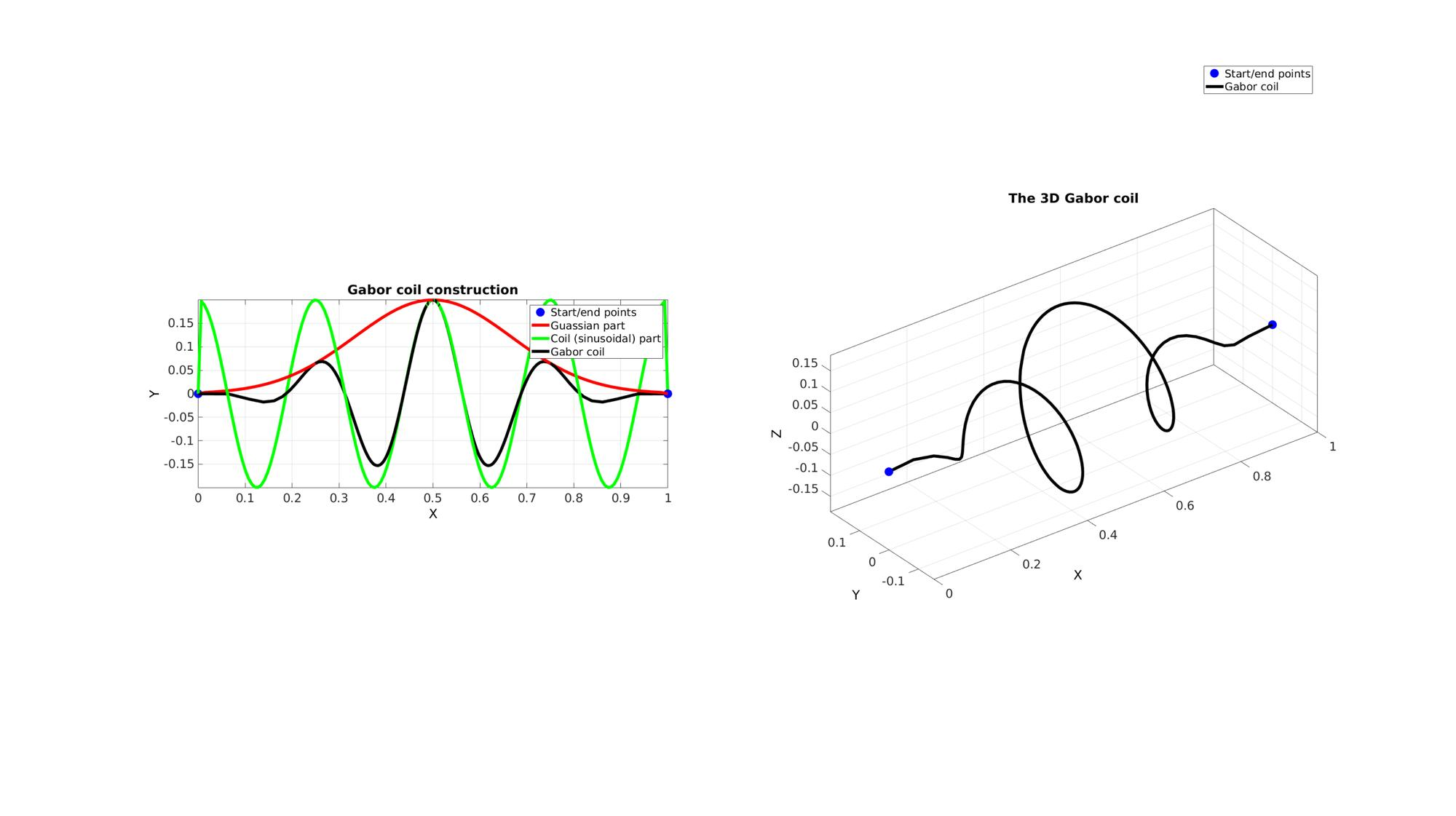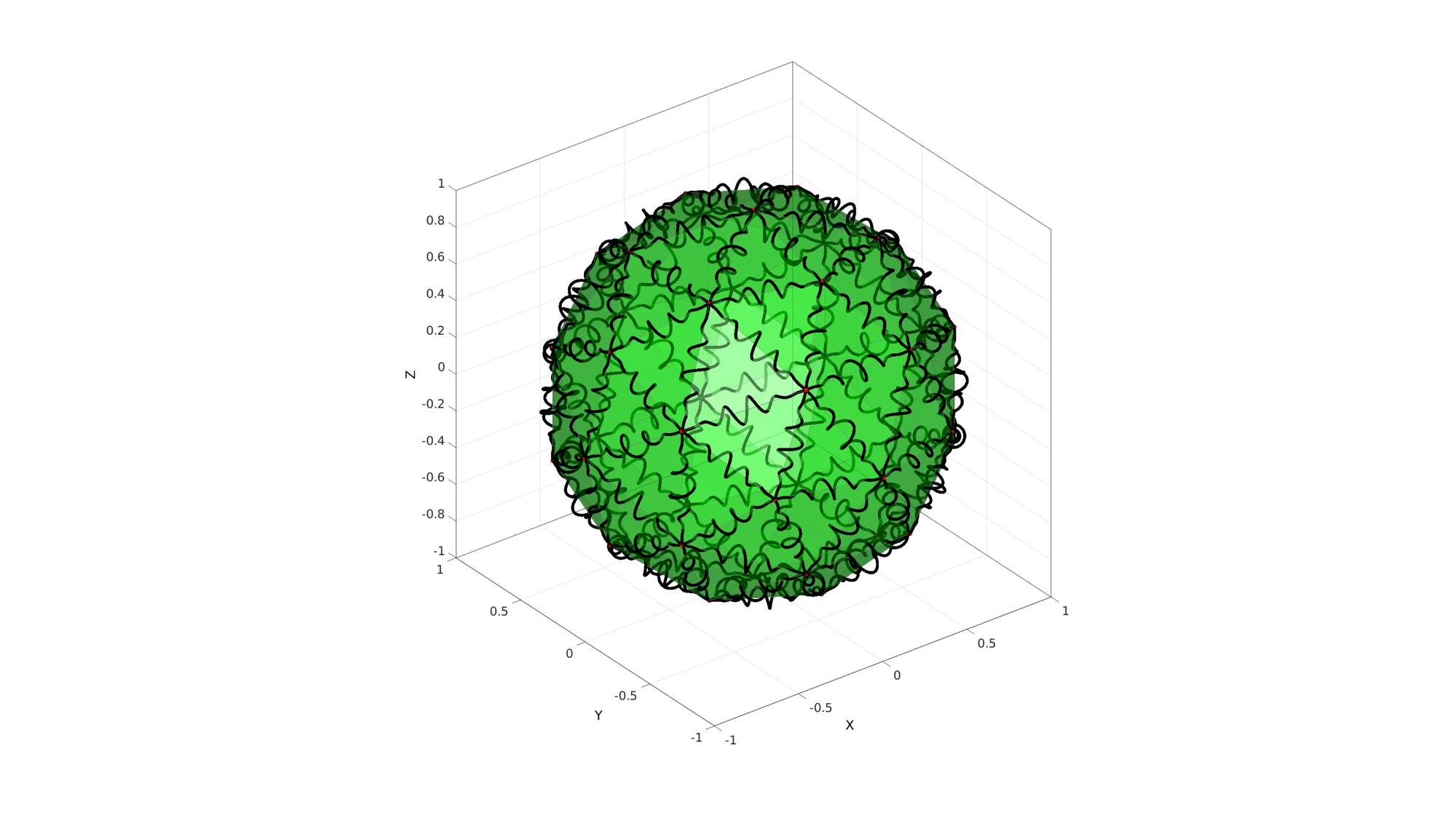# gaborCoil

Below is a demonstration of the features of the gaborCoil function

## Contents

```clear; close all; clc;
```

## Syntax

[V_coil_rep]=gaborCoil(varargin);

## Description

This function generates a so-called Gabor coil, i.e. a spiral which is modulated similar to a Gabor filter.

## Creating a single Gabor coil

```V=[0 0 0; 1 0 0];
E=[1 2];

%Create a gabor coil
optionStruct.numSteps=150; %Number of points used to define the curve.
optionStruct.numTwist=4; %Number of twists (for an unmodulated coil)
optionStruct.coilAmplitude=[]; %The coil amplitude (set empty to based on curve or edge length instead)
optionStruct.coilAmplitudeFactor=1/5; %The factor used to set local coil amplitude as a function of edge length
optionStruct.f=3; % The Gaussian bell curve will be truncated at n*the standard deviation on both sides.
optionStruct.funcMethod=2; %For loop based (1) or vectorised (2).
V_gabor_coil=gaborCoil(V,E,optionStruct);

%Get pure Gaussian part for illustration
optionStruct.numSteps=150; %Number of points used to define the curve.
optionStruct.numTwist=0; %Number of twists (for an unmodulated coil)
optionStruct.coilAmplitude=[]; %The coil amplitude (set empty to based on curve or edge length instead)
optionStruct.coilAmplitudeFactor=1/5; %The factor used to set local coil amplitude as a function of edge length
optionStruct.f=3; % The Gaussian bell curve will be truncated at n*the standard deviation on both sides.
optionStruct.funcMethod=2; %For loop based (1) or vectorised (2).
V_gauss=gaborCoil(V,E,optionStruct);

%Get pure coil part for illustration
optionStruct.numSteps=150; %Number of points used to define the curve.
optionStruct.numTwist=4; %Number of twists (for an unmodulated coil)
optionStruct.coilAmplitude=[]; %The coil amplitude (set empty to based on curve or edge length instead)
optionStruct.coilAmplitudeFactor=1/5; %The factor used to set local coil amplitude as a function of edge length
optionStruct.f=0; % The Gaussian bell curve will be truncated at n*the standard deviation on both sides.
optionStruct.funcMethod=2; %For loop based (1) or vectorised (2).
V_coil=gaborCoil(V,E,optionStruct);
```

Visualizing coil curve

```cFigure;
subplot(1,2,1);
title('Gabor coil construction');
hold on;
hp(1)=plotV(V,'b.','markerSize',50);
hp(2)=plotV(V_gauss,'r-','LineWidth',5);
hp(3)=plotV(V_coil,'g-','LineWidth',5);
hp(4)=plotV(V_gabor_coil,'k-','LineWidth',5);
legend(hp,'Start/end points','Guassian part','Coil (sinusoidal) part','Gabor coil');
axisGeom;
view(2);

subplot(1,2,2);
title('The 3D Gabor coil');
hold on;
hp2(1)=plotV(V,'b.','markerSize',50);
hp2(2)=plotV(V_gabor_coil,'k-','LineWidth',5);
legend(hp2,'Start/end points','Gabor coil');
axisGeom;

drawnow;
```## Creating Gabor coil on all edges in mesh

Creating example patch data

```[F,V]=geoSphere(1,1);
% [F,V]=stanford_bunny;
```

Get patch edges

```E=patchEdges(F,1);
```
```optionStruct.numSteps=150; %Number of points used to define the curve.
optionStruct.numTwist=5; %Number of twists (for an unmodulated coil)
optionStruct.coilAmplitude=[]; %The coil amplitude (set empty to based on curve or edge length instead)
optionStruct.coilAmplitudeFactor=1/10; %The factor used to set local coil amplitude as a function of edge length
optionStruct.f=3; % The Gaussian bell curve will be truncated at n*the standard deviation on both sides.
optionStruct.funcMethod=2; %For loop based (1) or vectorised (2).

[V_coil_rep]=gaborCoil(V,E,optionStruct);
```
```cFigure; hold on;
gpatch(F,V,'g',0.5*ones(1,3),0.5);
plotV(V,'r.','MarkerSize',25);
axisGeom;

for q=1:1:size(V_coil_rep,3)
plotV(V_coil_rep(:,:,q),'k-','lineWidth',5);
end

drawnow;
```GIBBON www.gibboncode.org

Kevin Mattheus Moerman, [email protected]

GIBBON footer text

GIBBON: The Geometry and Image-based Bioengineering add-On. A toolbox for image segmentation, image-based modeling, meshing, and finite element analysis.

Copyright (C) 2019 Kevin Mattheus Moerman

This program is free software: you can redistribute it and/or modify it under the terms of the GNU General Public License as published by the Free Software Foundation, either version 3 of the License, or (at your option) any later version.

This program is distributed in the hope that it will be useful, but WITHOUT ANY WARRANTY; without even the implied warranty of MERCHANTABILITY or FITNESS FOR A PARTICULAR PURPOSE. See the GNU General Public License for more details.

You should have received a copy of the GNU General Public License along with this program. If not, see http://www.gnu.org/licenses/.